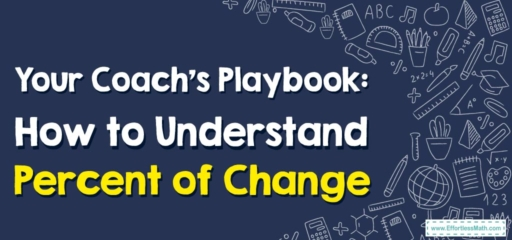# Your Coach’s Playbook: How to Understand Percent of Change

Hey there, Mathletes!

In today's training, we're going to tackle the percent of change – a handy tool that measures how much a quantity has changed relative to its original amount. But don't worry, as your friendly coach, I'll be guiding you every step of the way!## 1. Defining the Play: What is the Percent of Change?

Before we start the practice, let’s make sure we’re all on the same page:

• Percent of Change: This is a measure of how much a quantity has increased or decreased, given as a percentage of the original quantity. It’s like the scoreboard of change, helping us keep track of our progress!

## 2. The Game Plan: Calculating Percent of Change

Now that we understand what we’re dealing with, let’s go over the game plan for calculating the percent of change.

### Coach’s Playbook: Calculating Percent of Change

#### Step 1: Determine the Initial and Final Values

First off, we need to identify our initial (starting) and final (ending) values from the problem at hand.

#### Step 2: Calculate the Change

Next, find out how much the quantity has changed. You do this by subtracting the initial value from the final value.

#### Step 3: Divide the Change by the Initial Value

Now, find the ratio of the change to the initial value by dividing the amount of change by the initial value.

#### Step 4: Convert the Ratio to a Percentage

Finally, convert your answer to a percentage to get your percent of change. Just multiply the decimal ratio by $$100$$$$\%$$.

For example, if your team’s score increased from $$50$$ to $$75$$, what’s the percent of change?

1. Determine the Initial and Final Values: Your initial score is $$50$$, and your final score is $$75$$.
2. Calculate the Change: The change in score is $$75\ – 50 = 25$$.
3. Divide the Change by the Initial Value: The ratio of change to the initial value is $$\frac{25}{50} = 0.5$$.
4. Convert the Ratio to a Percentage: The percent of change is $$0.5\times100$$$$\%$$ $$= 50\%$$.

And there you have it, team! You’ve got the playbook for calculating the percent of change. Remember, math is just like any sport – the more you practice, the better you get. So, keep at it and you’ll be a pro in no time!

### What people say about "Your Coach’s Playbook: How to Understand Percent of Change - Effortless Math: We Help Students Learn to LOVE Mathematics"?

No one replied yet.

X
30% OFF

Limited time only!

Save Over 30%

SAVE $5 It was$16.99 now it is \$11.99